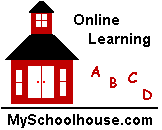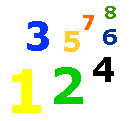# 1-50 Assessment

### Lesson List

Fill in the boxes with the symbols.

>  (greater than)

<  (less than)

=  (equals)

 6 + 2 3 + 2 6 + 1 2 + 8 7 - 1 9 - 29 + 0 10 + 0 10 - 5  6 - 3 6 - 5 7 - 77 + 1 9 - 1 8 + 2 10 + 0 2 + 4 6 + 03 + 2 1 + 4 8 - 2  3 + 3 2 + 1 8 - 26 - 2 2 + 2 3 - 1  7 - 5 5 + 5  6 + 2

Fill in the boxes.  Find the related fact family.

 1 + 0 = 1 + 1 = 1 1 + 1 = 2 - 1 = 12 + 1 = 3 + 2  = 3 3 - 1 = 2 3 - 2 =4 - 3 = 1 4 - = 3 3 + 1 = 4 1 + = 42 + 3 = 5 + 2 = 5 5 - 3 = 2 - 2 = 34 + 2 = 6 + 4 = 6 6 - 4 = 2 6 - 2 =

The 100 Table.

 1 2 3 4 5 6 7 8 9 10 11 12 13 14 15 16 17 18 19 20 21 22 23 24 25 26 27 28 29 30 31 32 33 34 35 36 37 38 39 40 41 42 43 44 45 46 47 48 49 50 51 52 53 54 55 56 57 58 59 60 61 62 63 64 65 66 67 68 69 70 71 72 73 74 75 76 77 78 79 80 81 82 83 84 85 86 87 88 89 90 91 92 93 94 95 96 97 98 99 100

Use the hundred chart to fill in the boxes below.

Find the number 12.  Count to find 10 more.

What number do you get?

Find the number 62.  Count to find 10 more.

What number do you get?

Find the number 84.  Count to find 10 more.

What number do you get?

Find the number 35.  What is 35 + 20?

Find the number 23.  What is 23 + 20?

Find the number 76.  What is 76 + 20?

Find the number 17.  What is 17 + 20?

Find the number 39.  What is 39 + 10?

Find the number 44.  What is 44 + 20?

Find the number 63.  What is 63 + 10?

Find the number 42.  What digit is in the tens place?

How many tens are in the number 42?

Find the number 71.  What digit is in the tens place?

How many tens are in the number 71?

Find the number 38.  What digit is in the tens place?

How many tens are in the number 38?

Find the number 99.  What digit is in the tens place?

How many tens are in the number 99?

Find the number 25.  What digit is in the tens place?

How many tens are in the number 25?

 Elementary & Middle School Lessons & Self-Correcting Tests for Children in all Subject Areas. If you have found an error or would like to make comments on this lesson, please email us at: MySchoolhouse.online@gmail.com Copyright 1999-2022 by Educational Designers, LLC.  All rights reserved. Lessons & Tests in Math, Reading, Spelling, Science, Language, and Social Studies. "This site uses cookies from Google to deliver its services and analyze traffic. Your IP address and user-agent are shared with Google along with performance and security metrics to ensure quality of service, generate usage statistics, and to detect and address abuse." WORK SPACE